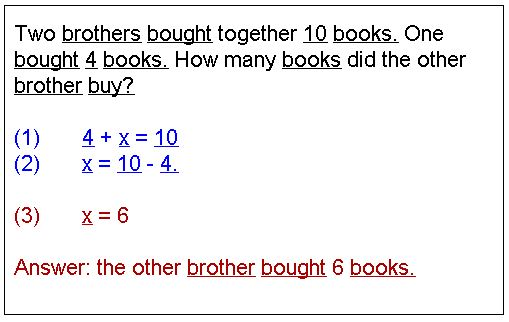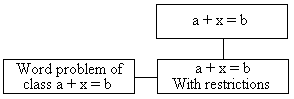# Word Problems That Lead to Simple Linear Equations

Word or story problems give us a first glimpse into how mathematics is used in the real word. To be solved, a word problem must be translated into the language of mathematics, where we use symbols for numbers - known or unknown, and for mathematical operations. When all is said and done, a word problem, stripped from inessential details, translates into one or more mathematical equations of one kind or another. After the equations have been solved, the result can be translated back into the ordinary language.

Mathematical language is clear and succinct. Mathematical formulation contains only what is important in a problem. But how does one know what is important and what is not, what is essential and what can be dispensed with? One simple approach to find out is to try changing the problem a small piece at a time. Play by ear: pay attention to those small modifications that do not distort the meaning of the problem.

As an example, let's start with one of the simplest problems. Every word and number shown in the applet is clickable. Numbers can be increased or decreased depending on whether you click a little to the right or to the left of their vertical center line.

// foreground color // background color // information pieces start with '@', followed by // a single letter from {v,a,f,e}, followed by ": ". // v - variable - anything that may change on a click // variables must precede all other pieces // f - formulation - word problem with embedded variables. // Many formulations are possible for a single problem. // Only one is shown at a time // e - equation - equation is like a formulation with a // potential provision to, e.g., enclose negative numbers // into parentheses // a - answer - like an equation, but allowed to have a parsable // portion. Parsables are written in the reverse Polish notations // with variables and operations separated by a comma // d - directive // define color, skip line // // whatever it is, a variable can be of the three types: // i = integer - on click changes up (right of center) and down (left of center) // a = attribute - any clickable and changeable word // v = variable - like an attribute but without related attributes // all 'name's below are 1 letter from [A-Ba-b1-0] // i, name, initial value, min, max // a, name, related attribute, list of values // v, name, list of symbols // formulations // equations // answers

### If you are reading this, your browser is not set to run Java applets. Try IE11 or Safari and declare the site https://www.cut-the-knot.org as trusted in the Java setup.What if applet does not run?

We may now make several observations. It's not important who the persons at hand are: brothers, sisters, or monsters. Nor is it important whether they owned, or bought, or had the goods in question. The nature of the goods is also inessential. What is essential is that the problem is concerned with three quantities, two of which add up to the third one. One of the quantities is unknown.

It appears that the problem belongs to a whole class of similar problems: all problems in that class translate in the mathematical language into equation (1). Equation (1), in turn, also affords several observations.

Firstly, the equation contains an arithmetic operation - addition. This comes directly from the problem that mentions two quantities together. Secondly, the name for the unknown quantity is quite arbitrary. The only important thing is that we anticipate existence of a number that satisfies the conditions of the problem. Mathematicians found it convenient to give the unknown number a name. François Viète (1540-1603) introduced letters to denote unknowns and constant numbers in equations. Vowels named unknowns, consonants named constants. It was René Descartes (1596-1650) who first used letters at the end of the alphabet for unknowns. By now, Descartes' usage evolved into a well established tradition.

A symbol used to denote an unknown quantity (or the quantity itself) is called a variable. This does not sound right as there is nothing that actually varies. But this is another tradition, a part of mathematical culture.

The third observation relates to other parts of the equation. One of the two quantities on the left and the one on the right are given numbers. These constitute the problem's data, the given. To solve equation (1), we subtract the number on the left from both sides of the equation. This is one of the principles annunciated yet by Euclid more than 2000 years ago: if equals be subtracted from equals, the remainders are equal. The transformation leads to equation (2). The next step is to perform subtraction on the right. Which results in equation (3). Equation (3) has particularly simple form: the variable - the unknown - equals a constant. But this is exactly what we were looking for: the value of the unknown. The equation has been solved.

It is important to realize that steps (1)-(3) solve the equation regardless of specific values of the two constants that appear in the equation. Solving a given equation is a mathematical problem in its own right. Thus we may observe that the problem of solving equation (1) belongs to a certain class of problems that are all solved in the same manner, as shown in steps (1)-(3). The general equation of this class can be described as

 (4) a + x = b,

where x stands for a variable or the unknown quantity, but a and b, although not assigned any specific values, are thought of as constants, arbitrary but given. (Letters in the beginning of the alphabet are usually reserved for such usage. As with the variable names, this convention too originated with René Descartes.) Furthermore, the names "a" and "b" are as arbitrary as the constants themselves. The equation B + u = F describes exactly the same class of equations as (4). To solve (4), apply Euclid's principle to obtain

 (5) x = b - a,

and finally carry out subtraction to find the value for the unknown x.

We now have to check whether the number just obtained solves the original word problem. As a matter of fact, it may or may not solve the problem. For example, assume b = 5 and a = 7. Then x = -2 is the solution to equation (4). Does it solve the following problem?

 Two sisters bought together 5 books. One bought 7 books. How many books did the other sister buy?

Of course we must admit that buying -2 books does not make sense. As a result, although x = -2 solves the equation 7 + x = 5, it does not solve the word problem above. Plainly, the latter does not have a solution. This is quite obvious for such a simple problem. Indeed, we might have noticed at the outset that the word problem had no solutions. The situation is less obvious when we consider more complicated problems. It will pay off, by that time, to have developed useful problem solving habits.

The following diagram may help in understanding of relationships the problems we looked at stand to each other.The general problem class described by the equation a + x = b is specialized by another class that adds restrictions on the possible solution. The word problem is related to the latter class. Restrictions we encountered so far are implicit in the word problem formulation. Such restrictions are called semantic.

### Summary

1. When translating a word problem into the mathematical language seek the essential. Inessential can be modified without affecting the meaning of the problem.
2. A variable in an equation is just an unknown quantity. Its name is quite arbitrary.
3. Think of a problem class to which the given problem belongs.
4. An equation may be less restrictive than the original problem. Check the answer against the problem's background.### Word Problems

(There are many more word problems discussed and solved at this site. The math tutorial started here continues with a similar approach over several additional examples.)